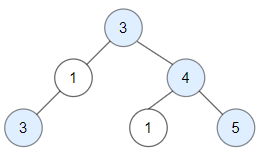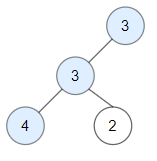# 1448. Count Good Nodes in Binary Tree

Medium
Given a binary tree `root`, a node X in the tree is named good if in the path from root to X there are no nodes with a value greater than X.
Return the number of good nodes in the binary tree.
Example 1:Input: root = [3,1,4,3,null,1,5]
Output: 4
Explanation: Nodes in blue are good.
Root Node (3) is always a good node.
Node 4 -> (3,4) is the maximum value in the path starting from the root.
Node 5 -> (3,4,5) is the maximum value in the path
Node 3 -> (3,1,3) is the maximum value in the path.
Example 2:Input: root = [3,3,null,4,2]
Output: 3
Explanation: Node 2 -> (3, 3, 2) is not good, because "3" is higher than it.
Example 3:
Input: root = 
Output: 1
Explanation: Root is considered as good.
Constraints:
• The number of nodes in the binary tree is in the range `[1, 10^5]`.
• Each node's value is between `[-10^4, 10^4]`.

### 解題

/**
* Definition for a binary tree node.
* type TreeNode struct {
* Val int
* Left *TreeNode
* Right *TreeNode
* }
*/
func goodNodes(root *TreeNode) int {
return helper(root, -100000)
}
func helper(root *TreeNode, m int) int {
if root == nil { return 0 }
if root.Val >= m {
return helper(root.Left, root.Val) + helper(root.Right, root.Val) + 1
}
return helper(root.Left, m) + helper(root.Right, m)
}
func max(a, b int) int {
if a > b { return a }
return b
}